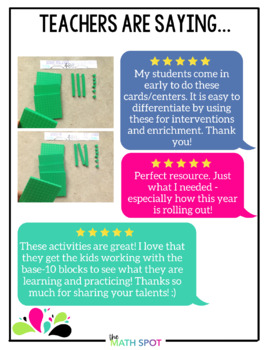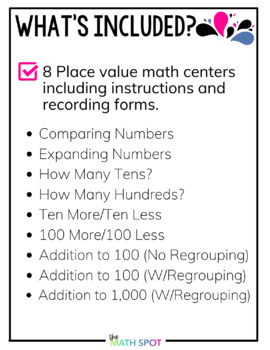# 2nd Grade Place Value Math Centers

Rated 4.81 out of 5, based on 101 reviews
101 Ratings;
2nd - 3rd
Subjects
Resource Type
Standards
Formats Included
• PDF
Pages
57 pages
Report this resource to TPT
##### Also included in
1. This bundle of math centers includes both hands on math centers to be used in a traditional math classroom as well as digital math centers for use with Google Classrooms. Included in this resource you will find: Traditional 2nd Grade Place Value Math CentersDigital 2nd Grade Place Value Math Centers
Price \$8.40Original Price \$12.00Save \$3.60

### Description

These 2nd grade place value math centers and math activities can be set up with nothing more than the math manipulatives you already have on hand! Your students will practice a variety of fun activities which will help your students to develop number sense and master place value and the 2nd Grade NBT standards.

Looking for a digital companion to these hands-on math centers? Save 30% when you buy the print and digital bundle of these math centers.

These centers are EASY to differentiate and recycle throughout the school year! Set any center out with linking cubes, base ten blocks, bundles of straws, place value disks or any other place value manipulative you love to use with your students!

Plus, every single center comes with a recording form so your students can stay on track.

Included in this pack are 9 place value games and activities designed to be used with hands-on manipulatives.

✨Comparing Numbers: 2.NBT.4 Students build and compare two 2-digit numbers.

✨Expanding Numbers: 2.NBT.1 &amp; 2.NBT.3 Students build a number on a place value mat and write in expanded form.

✨How Many Tens? 2.NBT.3 Given a number of ones, students build the number and determine the number of tens and left over ones.

✨How Many Hundreds? 2.NBT.3 Given a number of tens, students build the number and determine the number of hundreds and left over tens.

✨Ten More/Ten Less 2.NBT.8 Given a 2-digit number, students build the number and add or subtract ten sticks to determine the result.

✨100 More / 100 Less 2.NBT.8 Given a 3-digit number, students build the number and add or subtract 100 flats to determine the result.

✨**Place Value Addition to 100 2.NBT.5 Students decompose each addend into tens and ones in order to combine in a way which promotes place value understanding. This is the foundation for mental computation!

✨Addition to 100 2.NBT.5 Given two 2-digit numbers, students will build the two numbers and find the sum writing the problem in standard notation as they work.

✨Addition to 1000 2.NBT.7 Given two numbers, students will build the two numbers and find the sum.

Do you teach small group math intervention? These centers are a beautiful compliment to 2nd Grade Math Intervention Lessons!

Total Pages
57 pages
N/A
Teaching Duration
N/A
Report this resource to TPT
Reported resources will be reviewed by our team. Report this resource to let us know if this resource violates TPT’s content guidelines.

### Standards

to see state-specific standards (only available in the US).
Understand that the three digits of a three-digit number represent amounts of hundreds, tens, and ones; e.g., 706 equals 7 hundreds, 0 tens, and 6 ones. Understand the following as special cases:
Read and write numbers to 1000 using base-ten numerals, number names, and expanded form.
Compare two three-digit numbers based on meanings of the hundreds, tens, and ones digits, using >, =, and < symbols to record the results of comparisons.
Fluently add and subtract within 100 using strategies based on place value, properties of operations, and/or the relationship between addition and subtraction.
Add up to four two-digit numbers using strategies based on place value and properties of operations.